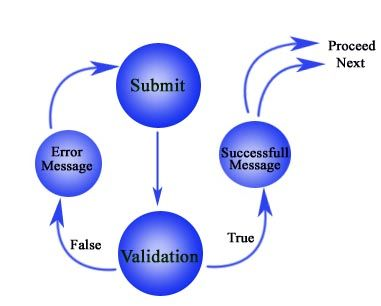# Education is not limited to just classrooms. It can be gained anytime, anywhere... - Ravi Ranjan (M.Tech-NIT)

## PHP Form validation

Last update on September 09 2017 06:08:52 (UTC/GMT +8 hours)

Preface

In this tutorial, we will see how to use PHP to validate the data collected from a form.

You will see how to validate various fields used in general, like text, list, checkbox, radio button and we will also see how to retain POST data, so that after the user submits the form, even if the data supplied is not valid, data is not lost.

Live Demo

Following is a live demo of the PHP form we will create by the end of this tutorial.

Pictorial presentation

The following picture shows what we need to do while validating a form.Base HTML file

1. <!DOCTYPE HTML>
2. <html lang="en">
3. <head>
4. <meta charset=utf-8>
5. <title>Form validation with parsely.js</title>
6. <link href="../../twitter-bootstrap/twitter-bootstrap-v2/docs/assets/css/bootstrap.css" rel="stylesheet">
7. <link href="parsely.css" rel="stylesheet">
8. <style type="text/css">
9. h1 {margin-bottom:20px}
10. input, label {margin-top:7px; margin-bottom:7px; color:#000066; font-size: 18px; padding-right: 7px}
11. input[type='checkbox'] {margin-left: 5px}
12. .note {color: #ff0000}
13. .success_msg{color:#006600}
14. </style>
15. </head>
16. <body>
17. <div class="container">
18. <h1>Travel reservation form</h1>
19. <h3><span class="note">*</span> denotes mandotory</h3>
20. <form id="registration_form" method="POST" action="<?php echo \$_SERVER['PHP_SELF']; ?>">
21.
22. </form>
23. </div>
24. </body>
25. </html>

We have used bootstrap for styling the form, but for a few styles we have added some custom styling.

We have used `POST` method and `action="<?php echo \$_SERVER['PHP_SELF']; ?>"` states that after submission form data will be handled by the PHP script present in this file only. You may opt for sending form data to a different file.

Validation for non-empty, alphabets and whitespace only

The following code is added within the form

1. <label>Full name<span class="note">*</span>:</label>
2.   <input type="text" name="full_name" placeholder="FirstName LastName" autofocus="autofocus" value="<?php echo \$_POST['full_name']; ?>">
3.   <?php echo "<p class='note'>".\$msg_name."</p>";?>
4.   <?php echo "<p class='note'>".\$msg2_name."</p>";?>

Code for validation

1. if (isset(\$_POST['submit'])) {
2. //checking name
3. if(emptyempty(\$_POST['full_name']))
4. \$msg_name = "You must supply your name";
5. \$name_subject = \$_POST['full_name'];
6. \$name_pattern = '/^[a-zA-Z ]*\$/';
7. preg_match(\$name_pattern\$name_subject\$name_matches);
8. if(!\$name_matches)
9. \$msg2_name = "Only alphabets and white space allowed";
10. }

email Validation

Code added within the form

1. <label>Email address<span class="note">*</span>:</label>
2.   <input type="text" name="email_addr" value="<?php echo \$_POST['email_addr']; ?>">
3.    <?php echo "<p class='note'>".\$msg_email."</p>";?>
4.   <?php echo "<p class='note'>".\$msg2_email."</p>";?>

Code for validation

1. if (isset(\$_POST['submit'])) {
2. //check email
3. if(emptyempty(\$_POST['email_addr']))
4. \$msg_email = "You must supply your email";
5. \$email_subject = \$_POST['email_addr'];
6. \$email_pattern = '/^w+([.-]?w+)*@w+([.-]?w+)*(.w{2,3})+\$/';
7. preg_match(\$email_pattern\$email_subject\$email_matches);
8. if(!\$email_matches)
9. \$msg2_email = "Must be of valid email format";
10. }

Selection list Validation

Code added within the form

1. <label>Select Tour Package<span class="note">*</span>:</label>
2.    <select name="package">
3.     <option value="Goa" <?= (\$_POST['package'] == "1")? "selected":"";?>>Goa</options>
4.     <option value="Kashmir" <?= (\$_POST['package'] == "2")? "selected":"";?>>Kashmir</options>
5.     <option value="Rajasthan" <?= (\$_POST['package'] == "3")? "selected":"";?>>Rajasthan</options>
6.    </select>

Code for validation

1. if (isset(\$_POST['submit'])) {
2. if(emptyempty(\$_POST['package']))
3. \$msg_package = "You must select a package";
4. }

Date Validation

Code added within the form

1. <label>Arrival date<span class="note">*</span>:</label>
2.   <input type="text" name="arv_dt" value="<?php echo \$_POST['arv_dt']; ?>">
3.   <?php echo "<p class='note'>".\$msg_dt."</p>";?>
4.   <?php echo "<p class='note'>".\$msg2_dt."</p>";?>
5.   <?php echo "<p class='note'>".\$msg3_dt."</p>";?>

Code for validation

1. if (isset(\$_POST['submit'])) {
2. //date validation
3. if(emptyempty(\$_POST['arv_dt']))
4. \$msg_dt = "You must supply an arival date";
5. if(!emptyempty(\$_POST['arv_dt']))
6. {
7.     \$dt = \$_POST['arv_dt'];
8.     \$array = explode("/",\$dt);
9.
10.     \$day = \$array;
11.     \$month = \$array;
12.     \$year = \$array;
13.
14.     if(!checkdate(\$month\$day\$year))
15.     {
16.     \$msg2_dt = "Must be in m/d/y format";
17.     }
18.     else
19.     {
20.     \$today = strtotime("now");
21.     if(strtotime(\$dt)<\$today)
22.     \$msg3_dt = "Date supplied is before present day";
23.     }
24. }
25. }

Validation for non-empty and non-negative integer

Code added within the form

1. <label>Number of persons<span class="note">*</span>:</label>
2.   <input type="text" name="persons" value="<?php echo \$_POST['persons']; ?>"s>
3.   <?php echo "<p class='note'>".\$msg_persons."</p>";?>
4.   <?php echo "<p class='note'>".\$msg2_persons."</p>";?>

Code for validation

1. if (isset(\$_POST['submit'])) {
2. //checking for non-empty and non-negative integer
3. if(emptyempty(\$_POST['persons']))
4. \$msg_persons = "You must supply number of persons travelling";
5. if(!emptyempty(\$_POST['persons']))
6. {
7. \$persons = \$_POST['persons'];
8. preg_match("@^([1-9][0-9]*)\$@"\$persons\$persons_match);
9. if(!\$persons_match)
10. \$msg2_persons = "Must be non negative integer";
11. }
12. }

Checkbox Validation

Code added within the form

1. <label>What would you want to avail?<span class="note">*</span></label>
2.  Boarding<input type="checkbox" name="facilities[]" value="boarding" <?php if(isset(\$_POST['submit']) && isset(\$_POST['facilities'])) echo "checked" ?> >
3.  Fooding<input type="checkbox" name="facilities[]" value="fooding" <?php if(isset(\$_POST['submit']) && isset(\$_POST['facilities'])) echo "checked" ?> >
4.  Sight seeing<input type="checkbox" name="facilities[]" value="sightseeing" <?php if(isset(\$_POST['submit']) && isset(\$_POST['facilities'])) echo "checked" ?> >
5.  <?php echo "<p class='note'>".\$msg_facilities."</p>";?>
6.  <?php echo "<p class='note'>".\$msg2_facilities."</p>";?>

Code for validation

1. if (isset(\$_POST['submit'])) {
2. //checking facilities
3. \$facilities = \$_POST['facilities'];
4.   if(emptyempty(\$facilities))
5.   {
6.     \$msg_facilities = "You must select facilities";
7.   }
8.
9.  if(!emptyempty(\$_POST['facilities'])) {
10.     \$no_checked = count(\$_POST['facilities']);
11.     if(\$no_checked<2)
12.     \$msg2_facilities = "Select at least two options";
13.     }
14. }

Validaiton for alphnumeric characters only with a minimum number of characters

Code added within the form

1. <label>Discout Coupon code:</label>
2.   <input type="text" name="dis_code" value="<?php echo \$_POST['dis_code']; ?>">
3.   <?php echo "<p class='note'>".\$msg_dis."</p>";?>
4.   <?php echo "<p class='note'>".\$msg2_dis."</p>";?>

Code for validation

1. if (isset(\$_POST['submit'])) {
2. //check discount coupon
3. //[^a-z0-9_]
4. if(\$_POST['dis_code'])
5. {
6.  \$dis_code = \$_POST['dis_code'];
7.  preg_match("/^[a-zA-Z0-9]+\$/"\$dis_code\$dis_match);
8.  if(!\$dis_match)
9.  \$msg_dis = "Must be alphanumric";
10.  if(strlen(\$dis_code)!='10')
11.  \$msg2_dis = "Must be 10 characters long";
12. }
13. }

Note that all the code snippets under "Code for validation" are wrapped by `if (isset(\$_POST['submit'])) `and `}`. In practice you may keep all of the codes within a single `if (isset(\$_POST['submit'])) `and `}`.

Validation for radio button

Code added within the form

1. <label>Terms and conditions<span class="note">*</span></label>
2.   <input type="radio" name="tnc" value="agree" <?php echo \$tncv; ?>>I agree<br>
3.   <input type="radio" name="tnc" value="disagree" <?php echo \$tnc1v; ?>>I disagree<br>
4.   <?php echo "<p class='note'>".\$msg_agree."</p>";?>
5.   <?php echo "<p class='note'>".\$msg2_agree."</p>";?>
1. //checking terms
2. \$tnc = \$_POST['tnc'];
3. switch(\$tnc)
4. {
5. case "agree":
6. \$tncv="checked";
7. \$tnc1v="";
8. break;
9.
10. case "disagree":
11. \$tncv="";
12. \$tnc1v="checked";
13. \$msg2_agree = "You must agree";
14. break;
15.
16. default// By default 1st option is selected
17. \$tncv="checked";
18. \$tnc1v="";
19. break;
20. };

Submit button

Code added within the form

1. <button type="submit" class="btn btn-large btn-primary" name="submit">Complete reservation</button>

Final piece of code to let the user know that form is validated

1. // validation complete
2. if(isset(\$_POST['submit'])){
3. if(\$msg_name=="" && \$msg2_name=="" && \$msg_email=="" && \$msg2_email=="" && \$msg_package=="" && \$msg_dt=="" && \$msg2_dt==""&& \$msg3_dt=="" && \$msg_persons=="" && \$msg2_persons=="" && \$msg_facilities=="" && \$msg2_facilities=="" && \$msg_dis=="" && \$msg2_dis=="" && \$msg_agree=="" && \$msg2_agree=="")
4. \$msg_success = "You filled this form up correctly";
5. }

And now you need to disaply a messge informing user that the form is validated. So, we add a single line of PHP code immediately before the form starts.

1. echo "<h3 class="success_msg">".\$msg_success."</h3>";

Download Source Code

How to Mail data collected after validation

IF you wish to send data collected through this form after validation to someone's mail address, following code would help you to do so.

1. // validation complete
2. if(isset(\$_POST['submit'])){
3. if(\$msg_name=="" && \$msg2_name=="" && \$msg_email=="" && \$msg2_email=="" && \$msg_package=="" && \$msg_dt=="" && \$msg2_dt==""&& \$msg3_dt=="" && \$msg_persons=="" && \$msg2_persons=="" && \$msg_facilities=="" && \$msg2_facilities=="" && \$msg_dis=="" && \$msg2_dis=="" && \$msg_agree=="" && \$msg2_agree=="")
4. \$msg_success = "You filled this form up correctly";
5. //send mail
6. \$to = "example@example.com";
7. \$subject = "Data collected thorugh from";
8. \$message = "<p>".\$_POST["full_name"]."</p><p>".\$_POST["email_addr"]."</p><p>".\$_POST['package']."</p><p>".\$_POST["arv_dt"]."</p><p>".\$_POST["persons"]."</p><p>".\$_POST["facilities"]."</p><p>".\$_POST["facilities"]."</p><p>".\$_POST["facilities"]."</p><p>".\$_POST["dis_code"]."</p><p>".\$_POST['tnc']."</p>";
9. \$from = "example2@example.com";
10. mail(\$to,\$subject,\$message);
11. }

So, if you try to understand how to write code for it step by step, step 1 is to declare the mail address to whom you want to send mail, step 2 is to write subject of the mail, step three is to collect data from POST so that you can write them to the body of mail, step 4 is to declare the mail id from which the mail is coming and finally sent mail with PHP mail() function. You may print some message after sending mail optionally